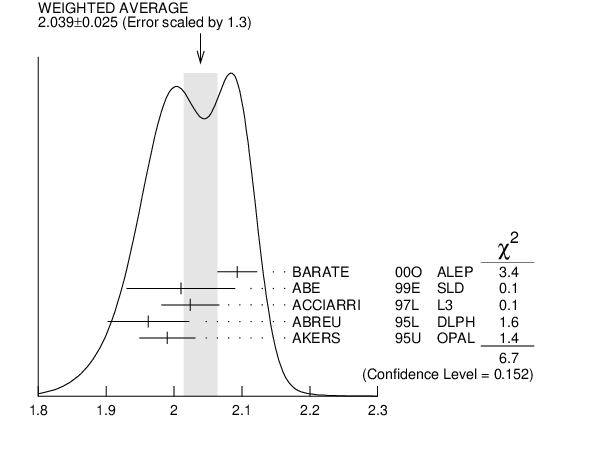# AVERAGE PARTICLE MULTIPLICITIES IN HADRONIC ${{\boldsymbol Z}}$ DECAY

Summed over particle and antiparticle, when appropriate.

# $\langle{}\boldsymbol N_{{{\boldsymbol K}^{0}}}\rangle{}$ INSPIRE search

VALUE DOCUMENT ID TECN  COMMENT
$\bf{ 2.039 \pm0.025}$ OUR AVERAGE  Error includes scale factor of 1.3.
$2.093$ $\pm0.004$ $\pm0.029$
 2000 O
ALEP ${\it{}E}^{\it{}ee}_{\rm{}cm}$= $91.2$ GeV
$2.01$ $\pm0.08$
 1999 E
SLD ${\it{}E}^{\it{}ee}_{\rm{}cm}$= $91.2$ GeV
$2.024$ $\pm0.006$ $\pm0.042$
 1997 L
L3 ${\it{}E}^{\it{}ee}_{\rm{}cm}$= $91.2$ GeV
$1.962$ $\pm0.022$ $\pm0.056$
 1995 L
DLPH ${\it{}E}^{\it{}ee}_{\rm{}cm}$= $91.2$ GeV
$1.99$ $\pm0.01$ $\pm0.04$
 1995 U
OPAL ${\it{}E}^{\it{}ee}_{\rm{}cm}$= $91.2$ GeV$\langle{}\mathit N_{{{\mathit K}^{0}}}\rangle{}$
References:
 BARATE 2000O
EPJ C16 613 Inclusive Production of ${{\mathit \pi}^{0}}$, ${{\mathit \eta}}$, ${{\mathit \eta}^{\,'}{(958)}}$, ${{\mathit K}_S^0}$ and ${{\mathit \Lambda}}$ in Two and Three Jet Events from Hadronic ${{\mathit Z}}$ Decays
 ABE 1999E
PR D59 052001 Production of ${{\mathit \pi}^{+}}$, ${{\mathit K}^{+}}$, ${{\mathit K}^{0}}$, ${{\mathit K}^{*0}}$, ${{\mathit \phi}}$, ${{\mathit p}}$ and ${{\mathit \Lambda}^{0}}$ in Hadronic ${{\mathit Z}}$ Decays
 ACCIARRI 1997L
PL B407 389 ${{\mathit K}_S^0}$ and ${{\mathit \Lambda}}$ Production in Quark and Gluon Jets at LEP
 ABREU 1995L
ZPHY C65 587 Production Characteristics of ${{\mathit K}^{0}}$ and Light Meson Resonances in Hadronic Decays of the ${{\mathit Z}^{0}}$
 AKERS 1995U
ZPHY C67 389 The Production of Neutral Kaons in ${{\mathit Z}^{0}}$ Decays and their Bose-Einstein Correlations